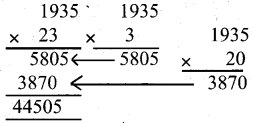# KSEEB Solutions for Class 5 Maths Chapter 1 Multiplication

Students can Download Maths Chapter 1 Multiplication Questions and Answers, Summary, Notes Pdf, KSEEB Solutions for Class 5 Maths helps you to revise the complete Karnataka State Board Syllabus and score more marks in your examinations.

## Karnataka State Syllabus Class 5 Maths Chapter 1 Multiplication

### KSEEB Class 5 Maths Multiplication Revision Exercise

I. Find the product of the following:

1. 35 × 0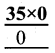2. 164 × 1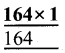3. 100 × 0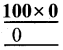4. 1 × 235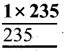5. 0 × 1,001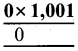6. 1 × 55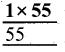II. Find the product of the following:

1. 45 × 16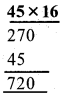2. 63 × 31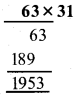3. 162 × 17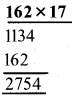4. 18 × 42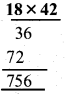5. 83 × 17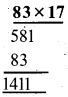6. 234 × 22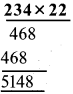III. Solve the following problems:

Question 1.
Cost of one notebook is Rs. 16, What is the cost of 12 notebooks?
Answer:
Cost of 1 notebook = Rs. 16
Cost of 12 notebooks = 16 × 12 = 192Question 2.
The school fee of a fifth class student is Rs. 38. What is the total fee of 128 students?
Answer:
Fee of a 5th class student = Rs 38
The total fee of student = 128 × 38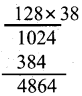total amount of 128 Rs.

Question 3.
63 students went on one-day excursion with their class teacher. The class teacher collected Rs. 150 from each student towards travel and lunch expenses. What is the total amount collected?
Answer:
The class teacher collects for each student Rs 150
The total number of students = 63 × 150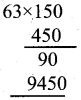Question 4.
The cost of a bag containing 10 kg. rice is Rs. 285. Find the cost of 25 bags of rice.
Answer:
The cost of a bay containing 10 kg rice Rs 85
The cost of 25 bays of rice = 285 × 10 = 2850 Rs

Question 5.
The headmaster decides to give first and second prize for various competitions. 16 students got first prize and 16 students got second prize. If the cost of first and second prize is Rs. 11 and Rs. 9 respectively, find the total amound required by headmaster to buy the prizes.
Answer:
The cost of First prise = Rs 11
16 students got First Prize = 16 × 11 = 170
The cost of second prize = Rs 9
16 students got second prize = 16 × 9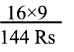The total amount required by headmaster to buy the prizes = 176 + 144 = Rs 320

### KSEEB Class 5 Maths Multiplication Ex 1.1

I. Multiply:

1. 146 × 173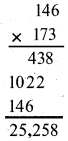2. 178 × 142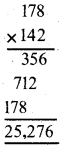3. 163 × 134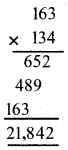4. 183 × 208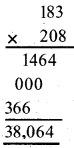5. 150 × 107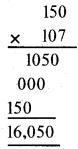6. 248 × 212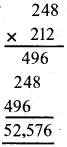7. 316 × 100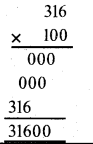8. 100 × 523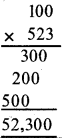II. Multiply:

1. 2,861 × 9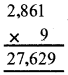2. 3,947 × 7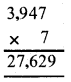3. 7,254 × 6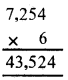4. 6,041 × 5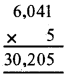5. 8,028 × 8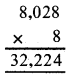6. 4,395 × 9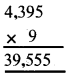III. Find the product:

1. 9,025 × 10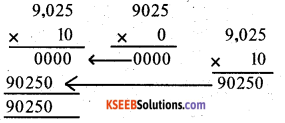2. 2,549 × 20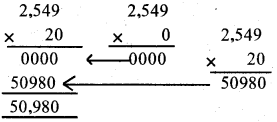3. 3,746 × 23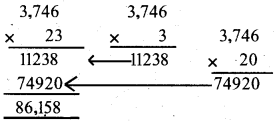4. 4,756 × 19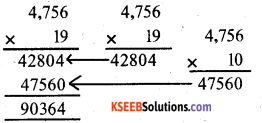5. 2,439 × 31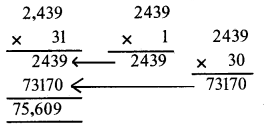6. 1,258 × 13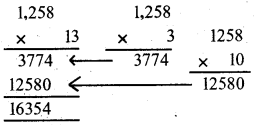7. 1,028 × 37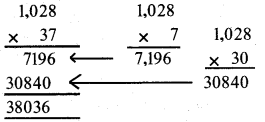8. 2,375 × 16

v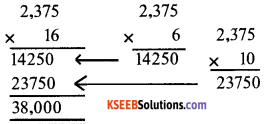IV. Multiply:

1. 21,497 × 3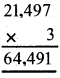2. 15,746 × 5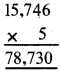3. 11,048 × 7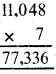4. 10,039 × 9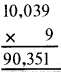V. Solve the following problems:

Question 1.
Mr. Siddappa is a fruit selling agent who e×port oranges. One bo× contains 144 oranges. If he export 259 boxes of oranges, find the total number of oranges exported.
Answer:
A box contains 144 oranges Mr. siddappa export 259 boxes of oranges
The total number of oranges Export 144 × 259 = 37,296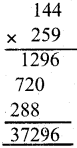Question 2.
In a protected forest area, 160 trees and planted in each row and 108 trees in each column. Find the total number of trees planted in the forest area.
Answer:
Trees are planted in each row 160
Trees are planted in each column 108
The total number of trees planted in the forest area = 160 × 108 = 17,280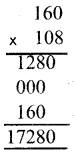Question 3.
A dealer buys 37 bicycles for his showroom: Cost of each bicycle is Rs.2,067. Find the amount paid by the dealer.
Answer:
The cost of each bicycle Rs 2067
A dealer buys 27 bicycles
The amount paid by the dealer = 2,067 × 27 = 55,809 Rs.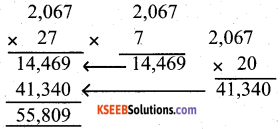Question 4.
A farmer grew 23 quintals of wheat. He sold them for Rs. 1,935 per quintal. How much amount did the farmer get by selling wheat?
Answer:
One quintal of wheat = Rs 1,935
A farmer grew 23 quiltals of wheat = 23
The farmer get by selling wheat = 1,935 × 23 = Rs 44,505